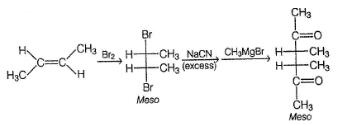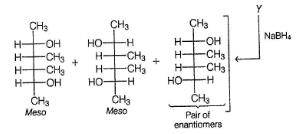Courses

# Test: Preparation Of Aldehydes And Ketones

## 25 Questions MCQ Test Chemistry Class 12 | Test: Preparation Of Aldehydes And Ketones

Description
This mock test of Test: Preparation Of Aldehydes And Ketones for Class 12 helps you for every Class 12 entrance exam. This contains 25 Multiple Choice Questions for Class 12 Test: Preparation Of Aldehydes And Ketones (mcq) to study with solutions a complete question bank. The solved questions answers in this Test: Preparation Of Aldehydes And Ketones quiz give you a good mix of easy questions and tough questions. Class 12 students definitely take this Test: Preparation Of Aldehydes And Ketones exercise for a better result in the exam. You can find other Test: Preparation Of Aldehydes And Ketones extra questions, long questions & short questions for Class 12 on EduRev as well by searching above.
QUESTION: 1

### Only One Option Correct Type Direction (Q. Nos. 1-12) This section contains 12 multiple choice questions. Each question has four choices (a), (b), (c) and (d), out of which ONLY ONE is correct. Q.  An optically active organic compound has molecular formula C5H12O(X). X on oxidation with CrO3/H2SO4 gives an achiral C5H10O. Hence, X could be

Solution:

Oxidation of X giving ketone as well as X is chiral, it must be a secondary alcohol with a-chiral carbon.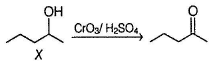QUESTION: 2

### Which of the following reaction will not produce an aldehyde?

Solution:

Anti vicinal-diols do not undergo oxidative cleavage with HIO4.

QUESTION: 3

### Which reagent below cannot reduce an acid chloride to an aldehyde?

Solution:

Na /C2 H5OH further reduces aldehydes to alcohols.

QUESTION: 4

The incorrect statement regarding oxo process for synthesis of an aldehyde is

Solution:

In an oxo process, formylation (addition of H and CHO) of double bond takes place, hence ketones cannot be synthesised in direct oxo process.

QUESTION: 5

All of the following reaction gives atleast one ketone as a significant organic product except

Solution:

It gives aldehydes as major product.

QUESTION: 6

All of the following results in the formation of an aldehyde except

Solution:

It gives a ketone.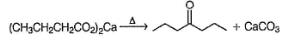QUESTION: 7

Consider the following reaction,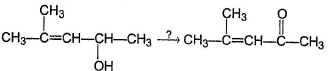All of the following reagents can bring about the above transformation except

Solution:

Alkaline permanganate (Baeyer’s reagent) also oxidises olefinic bonds to syn vicinal-diols.

QUESTION: 8

A hydrocarbon X(C7H12) on ozonolysis followed by the treatment with (CH3)2S gives C7H12O2 which gives positive Tollen’s test as well as positive iodoform test. The compoppd below satisfying the criteria of X is

Solution:

The ozonolysis product of X is a dicarbonyl which contains both aldehyde group and CH3CO— group.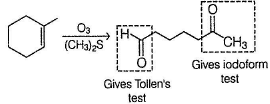QUESTION: 9

A hydrocarbon X has molecular formula C5H10 X on treatment with B2H6 in H2O2 /NaOH gives an optically active C5H12O which on treatment with CrO3/HCI / pyridine gives C5H10O which is still chiral. Which of the following can be a product of reductive ozonolysis of X?

Solution: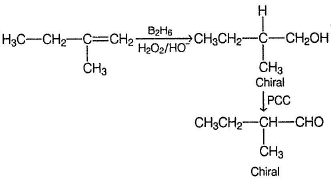QUESTION: 10

What is the final major product of the following reaction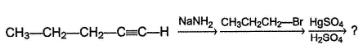Solution: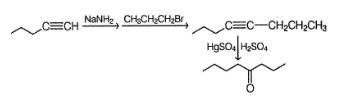QUESTION: 11

Consider the following reaction,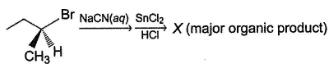(A pure enantiomer)

Q.

The incorrect statement regarding X is

Solution:

X will be a pure enantiomer of aldehyde.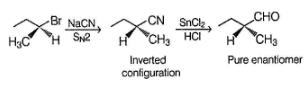QUESTION: 12

Consider the following reaction sequence,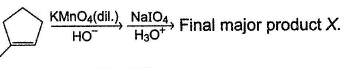Q.

The correct statement regarding X is

Solution: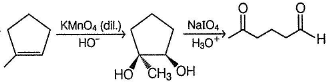*Multiple options can be correct
QUESTION: 13

One or More than One Options Correct Type

Direction (Q. Nos. 13-17) This section contains 5 multiple choice questions. Each question has four choices (a), (b), (c) and (dj, out of which ONE or MORE THAN ONE are correct.

Q.

In which of the following reactions, an aldehyde is formed as major product?

Solution:

(b) In option (b), aldehyde is not formed. When a terminal alkyne is oxidised with KMnO4, a carboxylic acid is always formed.
All options are preparation of aldehydes.

*Multiple options can be correct
QUESTION: 14

In which of the following reactions, ketone is formed as the major organic product?

Solution:

If acid derivatives like nitrile, acid chlorid e or ester is taken in excess in Grignard synthesis, second addition of Grignard’s reagent on carbonyl product does not succeed and carbonyls are obtained as major products.
In option (b), carboxylic acids and in option (d), an aldehyde is formed.

*Multiple options can be correct
QUESTION: 15

Consider the following reaction,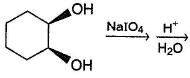Q.

The correct statement(s) regarding the above reaction is/are

Solution: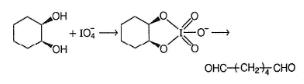Trans diastereomer of the above diol does not react due to its inability to form cyclic intermediate.

*Multiple options can be correct
QUESTION: 16

Consider the reaction mentioned below,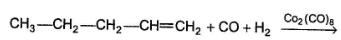Q.

The expected organic product(s) is/are

Solution:

Formylation of double bond (oxo process) occurs. However, the reaction is not regioselective, hence both isomers of aldehyde are formed.

*Multiple options can be correct
QUESTION: 17

Consider the following reaction,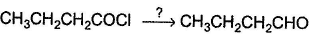Q.

Reagent(s) that can bring about the above reaction successfully is/are

Solution:

Both Rosenmund reduction (Pd/BaSO4)and reduction with Li[{(CH3)CO}3AIH] at - 80°C are effective in reducing acid chlorides into aldehydes.

QUESTION: 18

Comprehension Type

Direction (Q. Nos. 18-20) This section contains a paragraph, describing theory, experiments, data, etc. Three questions related to the paragraph have been given. Each question has only one correct answer among the four given options (a), (b), (c) and (d).

Passage

A hydrocarbon A (C10H18) is capable of showing both enantiomerism as well as diastereomerism. Treatment of A either with HgSO4 / H2SO4 or B2H/ H2O2 -NaOH results in the same carbonyl compound B. Also,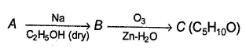C can also be obtained as one of the product in the following reaction.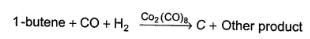Q.

What is the most likely structure of B?

Solution:

Compound A has a triple bond and it is symmetrical because its partially reduced product B gives single ozonolysis product C, Also, A shows both enantiomerism and diastereomerism, it must be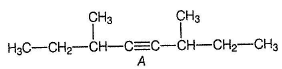As shown above, A has two chiral carbons but simultaneously, it is symmetrical. Hence, it has both meso and a pair of enantiomers as stereoisomers.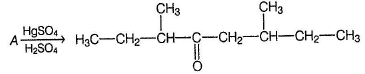QUESTION: 19

A hydrocarbon A (C10H18) is capable of showing both enantiomerism as well as diastereomerism. Treatment of A either with HgSO4 / H2SO4 or B2H/ H2O2 -NaOH results in the same carbonyl compound B. Also,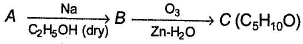C can also be obtained as one of the product in the following reaction.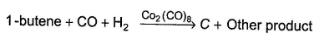Q.

What is the structure of compound C?

Solution:

Compound A has a triple bond and it is symmetrical because its partially reduced product B gives single ozonolysis product C, Also, A shows both enantiomerism and diastereomerism, it must beAs shown above, A has two chiral carbons but simultaneously, it is symmetrical. Hence, it has both meso and a pair of enantiomers as stereoisomers.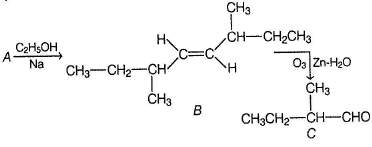QUESTION: 20

A hydrocarbon A (C10H18) is capable of showing both enantiomerism as well as diastereomerism. Treatment of A either with HgSO4 / H2SO4 or B2H/ H2O2 -NaOH results in the same carbonyl compound B. Also,C can also be obtained as one of the product in the following reaction.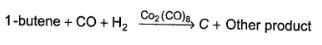Consider the reaction given below,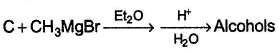Q.

How many different alcohols are expected?

Solution:

Compound A has a triple bond and it is symmetrical because its partially reduced product B gives single ozonolysis product C, Also, A shows both enantiomerism and diastereomerism, it must beAs shown above, A has two chiral carbons but simultaneously, it is symmetrical. Hence, it has both meso and a pair of enantiomers as stereoisomers.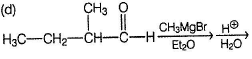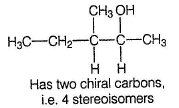*Answer can only contain numeric values
QUESTION: 21

One Integer Value Correct Type

Direction (Q. Nos. 21-25) This section contains 5 questions. When worked out will result in an integer from 0 to 9 (both inclusive).

Q.

How many different aldehyde isomers exist for C5H10O ?

Solution: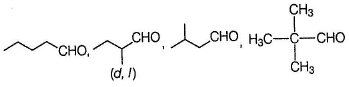*Answer can only contain numeric values
QUESTION: 22

How many different ketones isomer exist for C6H12O ?

Solution: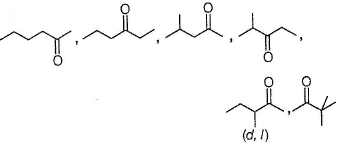*Answer can only contain numeric values
QUESTION: 23

How many different alcohol isomers with molecular formula C5H12O can be oxidised to ketones using K2Cr2O7 - H2SO4?

Solution:

All secondary alcohol isomers can be oxidised to ketones.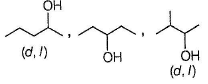*Answer can only contain numeric values
QUESTION: 24

If all the ketone isomers of C6H10O are reduced independently with NaBH4 , how many of them will produce racemic mixture of alcohols?

Solution: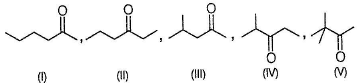All the above shown ketones on reduction with NaBH4 will produce racemic mixtures of alcohols. The isomer below already has a chiral carbon, show enantiomerism. A pure enantiomer of this ketone, on reduction with NaBH4, will produce pair of diastereomers.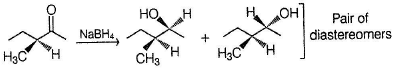*Answer can only contain numeric values
QUESTION: 25

Consider the following reaction,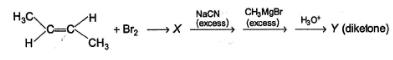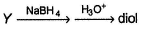Q.

How many different diols are expected at the end of the above reaction?

Solution: